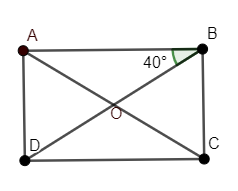Courses
Courses for Kids
Free study material
Free LIVE classes
MoreLIVE
Join Vedantu’s FREE Mastercalss

# ABCD is a rectangle, where AC and BD are diagonals,$\angle ABD=40{}^\circ$, determine $\angle DBC$.Verified
360.6k+ views
Hint: Use the basic property of a rectangle that can be given as “All the angles of a rectangle are equal to each other and each of them is a right angle i.e. $90{}^\circ$.”

Here, we have a rectangle ABCD with $\angle ABD=40{}^\circ$ where AC and BD are diagonals and we need to determine $\angle DBC$.
Now, let us draw a diagram representing all the given information from the problem, we getWhere ‘O’ is the intersection point of AC and BD.
Now, we need to use the fundamental property of the rectangle that can be given as “Each interior angle of the rectangle is a right angle i.e.$90{}^\circ$.”
Hence, we can write
$\angle A=\angle B=\angle C=\angle D=90{}^\circ \ldots \ldots (1)$
So, we can write equation between $\angle ABD$ and $\angle DBC$ as
$\angle B=\angle ABD+\angle DBC=90{}^\circ \ldots \ldots (2)$
It is given that $\angle ABD=40{}^\circ$. By putting value of $\angle ABD$ in equation (2), we get
$40{}^\circ +\angle DBC=90{}^\circ$
Subtracting $40{}^\circ$from both sides, we get
$40{}^\circ -40{}^\circ +\angle DBC=90{}^\circ -40{}^\circ$
or $\angle DBC=50{}^\circ$
Hence, if $\angle ABD=40{}^\circ$then $\angle DBC=50{}^\circ$in a rectangle ABCD.
So, Option B. is the correct answer.

Note: One can give $40{}^\circ$ as an answer because the rectangle looks symmetric when we cut it across the diagonal. Hence, one can think that $\angle ABD=\angle DBC=40{}^\circ$ which is wrong. So, take care of that.
Another approach for this question would be that we can calculate $\angle BDC$ as $40{}^\circ$ because $\angle ABD$ and $\angle BDC$ are alternate angles where AB||CD. Now, $\angle BCD=90{}^\circ$, and apply the property of triangle as “Sum of interior angles of triangle is $180{}^\circ$.” So,$\angle DBC+\angle BCD+\angle BDC=180{}^\circ$.
Last updated date: 21st Sep 2023
Total views: 360.6k
Views today: 11.60k